﻿ 基于AMF-HXA的长株潭空气质量指数互相关性分析

# 基于AMF-HXA的长株潭空气质量指数互相关性分析Cross-Correlation Analysis of Air Quality Index in Changsha, Zhuzhou and Xiangtan Based on AMH-HXA

Abstract: As the economy soars, air pollution sweeps across the China in recent years. Industrial waste gas and life stove and heating are two main sources of the air pollution. How to uncover the relationship between the air quality index (AQI) and traditional air pollution factors, such as industrial pollutants, NO2 and fine particles, such as PM2.5 for different cities has become a key point we care about. In this paper, we apply the popular analogous multifractal height cross-correlation analysis (AMF-HXA) into investigating the air quality in neighboring Changsha city, Zhuzhou city and Xiangtan city in Central China, which includes that the scale free of AQI, PM2.5 concentration and NO2 concentration, the fluctuation difference between the AQI and PM2.5 as well as NO2, respectively, the cross-correlation of the two pollution factors in each two cities, and the cross-correlations together with the significance between the AQI and above two pollution factors in each cities. The main conclusions are: 1) the fluctuation of AQI is consistent with that of PM2.5 concentration but disagreed with that of NO2 in different seasons; 2) there is high cross-correlation significance of PM2.5 between each two cities in all of seasons but the significance of the cross-correlation of NO2 is different in different seasons. These conclusions can provide a new insight to unveil the variation tendency of air pollution between different cities.

1. 引言

2. 方法与数据来源

2.1. 仿多重分形高度互相关分析(AMF-HXA)

$X\left(t\right)=\underset{i=1}{\overset{t}{\sum }}\left[{x}_{i}-〈x〉\right],\text{\hspace{0.17em}}Y\left(t\right)=\underset{i=1}{\overset{t}{\sum }}\left[{y}_{i}-〈y〉\right]$ (1)

${\Delta }_{L}X\left(t\right)Y\left(t\right)=\left[X\left(t\right)-X\left(t+L\right)\right]\cdot \left[Y\left(t\right)-Y\left(t+L\right)\right]$ (2)

${F}_{xy}^{q}\left(L\right)=\frac{1}{N-L}\underset{t=1}{\overset{N-L}{\sum }}\mathrm{sgn}\left({\Delta }_{L}X\left(t\right)Y\left(t\right)\right){|{\Delta }_{L}X\left(t\right)Y\left(t\right)|}^{q/2}$ (3)

${\left[{F}_{xy}^{q}\left(L\right)\right]}^{1/q}={F}_{xy}^{}\left(q,L\right)\propto {L}^{{\lambda }_{×}\left(q\right)}.$ (4)

${F}_{xx}^{}\left(q,L\right)={〈{\Delta }_{L}X{\left(t\right)}^{q}〉}^{1/q}\propto {L}^{{\gamma }_{xx}}$ (5)

$\Delta F\left(q,L\right)=\mathrm{log}{F}_{xx}\left(q,L\right)-\mathrm{log}{F}_{yy}\left(q,s\right)$ (6)

AMF-HXA方法能准确检测两个时间序列的互相关性，然而，它不能定量的刻画这种互相关性。为了定量描述互相关的程度，基于AHXA(即取q = 2时)，利用公式(3)得到的两个序列的协方差函数与由公式(5)得到的两个序列的方差函数求比值，得到了一种所谓的基于AHXA的互相关系数，如下所示：

$\rho \left(L\right)=\frac{{F}_{xy}^{2}\left(L\right)}{{F}_{xx}\left(2,L\right){F}_{yy}\left(2,L\right)}=\frac{〈sign\left({\Delta }_{L}X\left(t\right)Y\left(t\right)\right)\cdot |{\Delta }_{L}X\left(t\right)Y\left(t\right)|〉}{\sqrt{〈{\Delta }_{L}X{\left(t\right)}^{2}〉\cdot 〈{\Delta }_{L}Y{\left(t\right)}^{2}〉}}$ (7)

$\rho \left(L\right)$ 是一个无量纲量，且满足 $-1\le \rho \left(L\right)\le 1$

2.2. 数据来源

3. 数据分析与讨论

3.1. 互相关波动函数幂律关系检测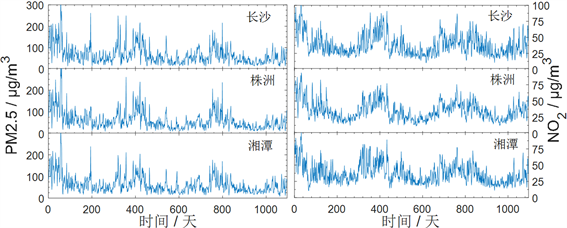Figure 1. The PM 2.5 index and NO2 index of Changsha, Zhuzhou and Xiangtan from Dec. 1, 2013 to Nov. 30, 2016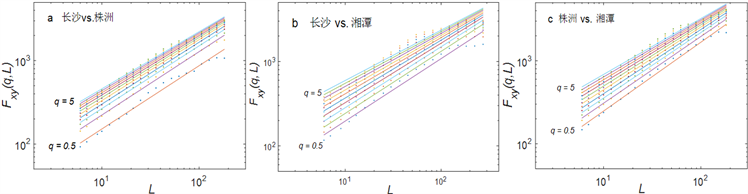Figure 2. Multifractal nature in the power-law of dependence of Fxy(q, L) on L for PM2.5 concentration: (a) is for Changsha vs. Zhuzhou: (b) is for Changsha vs. Xiangtan; and (c) is for Zhuzhou vs. Xiangtan

3.2. 空气质量指数与污染因子的关系Figure 3. Difference of fluctuation functions between AQI and the two pollution factors, namely, PM2.5 concentration (a and b) and NO2 concentration (c and d) of Changsha. The left two panels are for smaller amplitude fluctuations and the right two panels are for larger amplitude fluctuations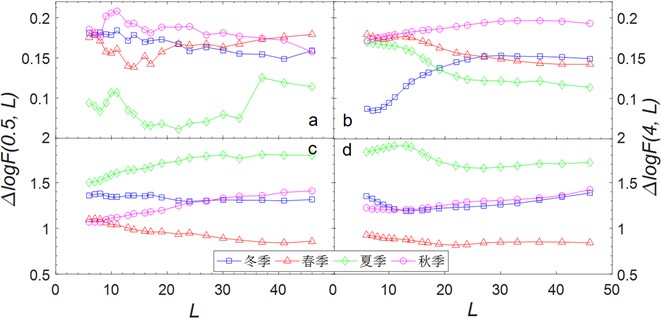Figure 4. Difference of fluctuation functions between AQI and the two pollution factors, namely, PM2.5 concentration (a and b) and NO2 concentration (c and d) of Zhuzhou. The left two panels are for smaller amplitude fluctuations and the right two panels are for larger amplitude fluctuations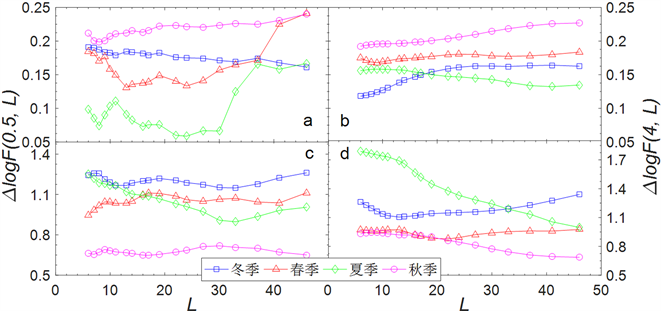Figure 5. Difference of fluctuation functions between AQI and the two pollution factors, namely, PM2.5 concentration (a and b) and NO2 concentration (c and d) of Xiangtan. The left two panels are for smaller amplitude fluctuations and the right two panels are for larger amplitude fluctuations

3.3. 长株潭三城市间空气污染因子的相关性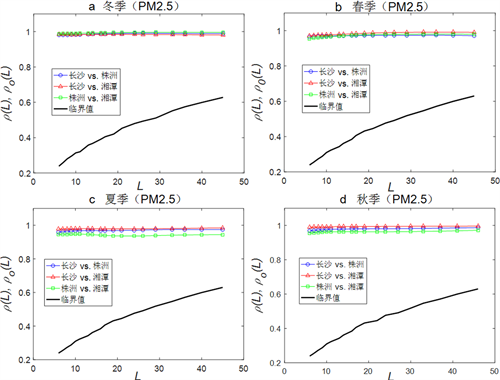Figure 6. Cross-correlation coefficients and their significances of PM2.5 concentration among Changsha, Zhuzhou and Xiangtan: (a), (b), (c) and (d) denote winter, spring, summer and fall, respectively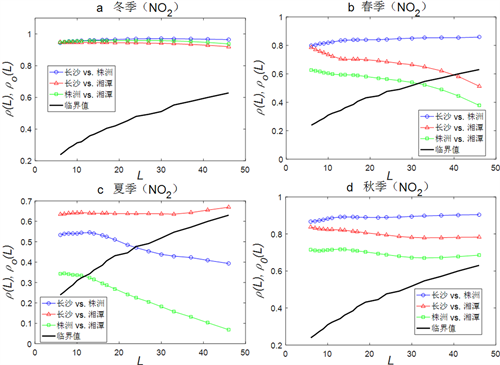Figure 7. Cross-correlation coefficients and their significances of NO2 concentration among Changsha, Zhuzhou and Xiangtan: (a), (b), (c) and (d) denote winter, spring, summer and fall, respectively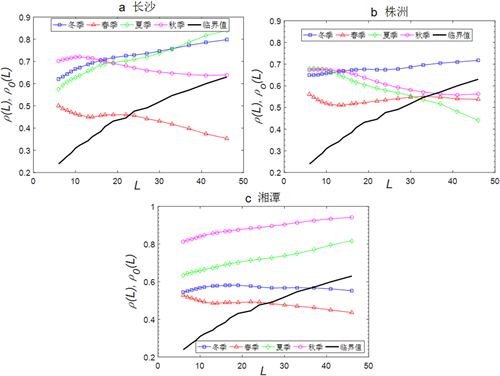Figure 8. Cross-correlation coefficients and their significances between the PM2.5 and NO2 of winter, spring, summer and fall for the three studied cities: (a), (b) and (c) denote Changsha, Zhuzhou and Xiangtan, respectively

4. 结语

 Chan, C. and Yao, X. (2008) Air Pollution in Mega Cities in China. Atmospheric Environment, 42, 1-42.
https://doi.org/10.1016/j.atmosenv.2007.09.003

 Han, L., Zhou, W. and Li, W. (2015) City as a Major Source Area of Fine Particulate (PM 2.5) in China. Environmental Pollution, 206, 183-187.
https://doi.org/10.1016/j.envpol.2015.06.038

 Han, L., et al. (2015) Increasing Impact of Urban Fine Particles (PM2.5) on Areas Surrounding Chinese Cities. Scientific Reports, 5, Article ID: 12467.
https://doi.org/10.1038/srep12467

 Shen, C., Li, C. and Si, Y. (2015) A Detrended Cross-Correlation Analysis of Meteorological and API Data in Nanjing, China. Physica A, 419, 417-428.
https://doi.org/10.1016/j.physa.2014.10.058

 Shen, C. and Li, C. (2016) An Analysis of the Intrinsic Cross-Correlations between API and Meteorological Elements Using DPCCA. Physica A, 446, 100-109.
https://doi.org/10.1016/j.physa.2015.11.024

 Du Kang, D., Lee, D.I., Kwon, B.H., et al. (2013) Features of the Detrended Cross-Correlation Analysis in the Time Series between Absorbable Particulate Matter and Meteorological Factors. Journal of the Korean Physical Society, 63, 10-17.
https://doi.org/10.3938/jkps.63.10

 Podobnik, B. and Stanley, H.E. (2008) Detrended Cross-Correlation Analysis: A New Method for Analyzing Two Nonstationary Time Series. Physical Review Letters, 100, 84-102.
https://doi.org/10.1103/PhysRevLett.100.084102

 Podobnik, B, Jiang, Z.Q., Zhou, W.X, et al. (2011) Statistical Tests for Power-Law Cross-Correlated Processes. Physical Review E, 84, Article ID: 066118.
https://doi.org/10.1103/PhysRevE.84.066118

 Zebende, G.F. (2011) DCCA Cross-Correlation Coefficient: Quantifying Level of Cross-Correlation. Physica A, 390, 614-618.
https://doi.org/10.1016/j.physa.2010.10.022

 Barabási, A.L., Szépfa-lusy, P. and Vicsek, T. (1991) Multifractal Spectra of Multi-Affine Functions. Physica A, 178, 17-28.
https://doi.org/10.1016/0378-4371(91)90072-K

 Wang, F., Liao, G.P., Li, J.H., Zou, R.B. and Shi, W. (2013) Cross-Correlation Detection and Analysis for California’s Electricity Market Based on Analogous Multifractal Analysis. Chaos, 23, Article ID: 013129.
https://doi.org/10.1063/1.4793355

 Wang, F., Yang, Z. and Wang, L. (2016) Detecting and Quantifying Cross-Correlations by Analogous Multifractal Height Cross-Correlation Analysis. Physica A, 444, 954-962.
https://doi.org/10.1016/j.physa.2015.10.096

 Zhou, W.X., Sornette, D. and Yuan, W.K. (2006) Inverse Statistics and Multi-fractality of Exit Distances in 3D Fully Developed Turbulence. Physica D, 214, 55-62.
https://doi.org/10.1016/j.physd.2005.12.004

Top